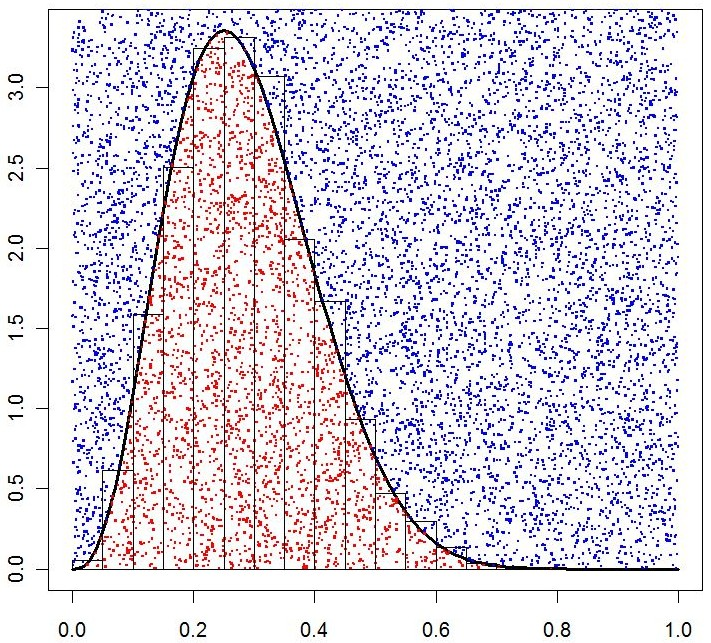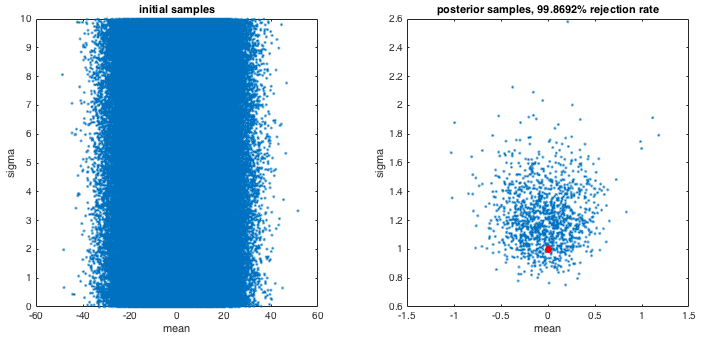I’ve been using MCMC, but I’ve wanted to flesh out my knowledge and explore the space of sampling approaches a little more. One very simple, yet inefficient method, is rejection sampling. Here is a little Matlab example I put together after seeing how easy it was.

 %% true probability distribution true_func = @(x) betapdf(x,1+1,1+10); %% Do rejection sampling % create many samples on interval 0-1 x_samples = rand(10^6,1); % evaluate for each sample sample_value = true_func(x_samples); % accept in proportion to highest max_value = max(sample_value); accepted = rand(10^6,1) < (sample_value/max_value); samples = x_samples(accepted); %% plot subplot(1,2,1) x = linspace(0,1,1000); plot(x, true_func(x) ) subplot(1,2,2) hist(samples,1000) proportion_rejected = sum(accepted==0) / numel(x_samples); title([num2str(proportion_rejected*100) '% of samples rejected']) % example inspired by http://www.r-bloggers.com/a-simple-explanation-of-rejection-sampling-in-r/

Which results in this output.So we have a good set of samples which well approximates the true distribution. A few notes:

• Generation of the samples (before rejection) must come from an appropriately broad prior which encompasses the relevant regions.
• If it is costly to evaluate the function for each sample, then this is inefficient because many of those samples will be subsequently thrown away.
• However, it is very very simple to code.

This is a nice figure to convey the intuition.## Worked example: parameter estimation

Now let’s use this approach in a toy example to estimate the mean and sigma of a Gaussian distribution, based upon 20 draws.

 true_mean = 0; true_sigma = 1; % likelihood_func = @(x, mean, sigma) normpdf(x, mean, sigma); % the above function to calcalate in matrix form, for speed likelihood_func = @(x, mean, sigma)… prod(normpdf(repmat(x,[1 numel(mean)]),… repmat(mean, [1 numel(x)])',… repmat(sigma,[1 numel(x)])' ), 1); %% generate data N=20; observed_data = normrnd(true_mean, true_sigma, [N 1]); %% Do rejection sampling % create many samples for mean and sigma n_samples = 10^6; mean_samples = (rand(n_samples,1)-0.5)*5; sigma_samples = rand(n_samples, 1) * 10; % evaluate likelihood for each (mean, sigma) sample sample_value = likelihood_func(observed_data, mean_samples, sigma_samples); % accept in proportion to highest max_value = max(sample_value); accepted = rand(1,n_samples) < (sample_value./max_value); mean_samples_accepted = mean_samples(accepted); sigma_samples_accepted = sigma_samples(accepted); %% plot subplot(1,2,1) plot(mean_samples,sigma_samples,'.') xlabel('mean') ylabel('sigma') title('initial samples') subplot(1,2,2) plot(mean_samples_accepted,sigma_samples_accepted,'.') hold on plot(true_mean, true_sigma, 'r.','MarkerSize',5^2) xlabel('mean') ylabel('sigma') title(['posterior samples, ' num2str(sum(accepted==0)/n_samples*100)… '% rejection rate'])

The code above results in a reasonable set of samples from the posterior. However, note the exceptionally high rejection rate. This is because the proposal distribution is broad (note the axis scales) because in real situations we may have very little knowledge of where the posterior density is focussed.## Thoughts

Rejection sampling is easy to implement, but it is very inefficient. However, I can imagine some interesting schemes were you start off with many samples over a broad region of parameter space to get an initial indication of the region of parameter space of interest. You could then repeat rejection sampling with with the proposal distribution focussed upon this interesting region of parameter space.

## Remaining questions

• I am not sure if the proposal distribution has to be uniform.
• If not, does the proposal distribution represent our prior beliefs?

## TODO

update example based on insights in this great video

## Join the conversation

1.2.1.Richard Fisher says: